## Introduction

Prerequisites: Shortest Path, Priority Queue, Greedy Algorithm

Dijkstra's is a greedy approach to find the shortest path in a graph with positive weights. It has many useful applications in networking and it can be extended to a variety of problems.

Dijkstra works by beginning at the starting node repeatedly picking the next closest node of those already visited.

If Dijkstra's is implemented using a priority queue, the run time is O(n log n).

If a negative cycle exists within the graph, then the algorithm breaks as it will repeatedly try to take the negative edges. See the Bellman Ford algorithm for finding negative cycles in a graph.

A naive fix for negative cycles would be to offset all edges by the largest negative edge and then subtract it from the resulting total but this does not work. Consider an example where you have the path from A to B. The first path from A->B has a weight of 2 and the second path has weights 1,1,-2. Clearly, the second route has less cost. If we try to make the length positive by adding all costs by 2, we will have the first path of weight 4 and the second path of weights 3,3,0. From the adjusted weights, the first path minimizes the total cost which is incorrect.

In general, Dijkstra is usually the method for finding the minimum cost between two nodes in any kind of network. For example, Dijkstra can be used in computer networking to find the shortest path between two hosts. It can also be used in flight networking to find the cheapest cost to get from one airport to another airport.

## Implementation

At each node we visit we keep track of the minimum cost it takes to reach to reach that node from the starting node.

1. Start at the starting node.
2. Find an unvisited node that has the least cost to reach from the visited nodes.
3. Mark that node as visited.
4. Repeat until all nodes are visited.

When we reach a node for the first time, it will be the shortest path from the start node to that node. (Try to prove this to yourself).

We first start at the starting node. The distance from the starting node to the starting node is obviously 0.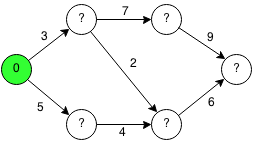From the starting node, we have two nodes we can reach. The top node has a cost of 3 to reach and the bottom node has a cost of 5 to reach.We pick the smallest node that can be reached and we mark it as visited. Once we visit a node, we can guarantee that it is the smallest cost to reach it. The next nodes that can be reached have minimum costs of 10, 5 and 5.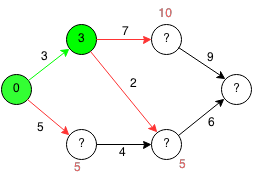We are indifferent to both 5's as they are both the minimum and we can choose either. We mark the node as visited and we find the minimum costs to other nodes which are 5,10,11.We take the next smallest which is 5 and we mark the node as visited. The next costs are 10 and 11.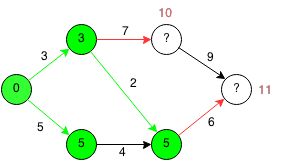We take the smallest which is 10 and we now only have one last node to reach at a cost of 11.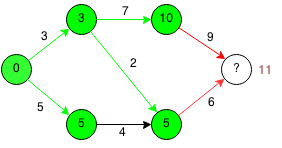Finally, we have the shortest path from the start node to the end node.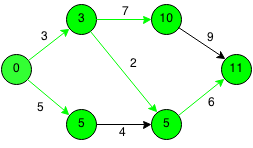### Code

We can implement Dijkstra's algorithm efficiently using a priority queue.

```class node implements Comparable<node> {
int weight, index;
public node(int weight, int index) {
this.weight = weight;
this.index = index;
}
public int compareTo(node e) {
return weight - e.weight;
}
}

public static int dijkstra(int[][] adjMatrix, int start, int end) {

PriorityQueue<node> pq = new PriorityQueue<node>();

// Initialize visited to false.
boolean visited[] = new boolean[n];
for (int i = 0; i < n; i++) {
visited[i] = false;
}

// Add the start node to the queue.

// Keep going until all nodes are visited or queue is empty.
while (!visited[end] && !pq.isEmpty()) {

// Get node with lowest total weight.
node curNode = pq.poll();

// Skip node is already visited.
if (visited[curNode.index]) {
continue;
}

// Mark node as visited.
visited[curNode.index] = true;

// If current node is end node then we are done.
if (curNode.index == end) {
return curNode.weight;
}

// Iterate through neighbors of current node.
for (int i = 0; i < n; i++) {
// Iterate through each unvisited neighbor.
if (adjMatrix[curNode.index][i] > 0 && !visited[i]) {
// Set add edge weight to current weight.
int newWeight = curNode.weight + adjMatrix[curNode.index][i];